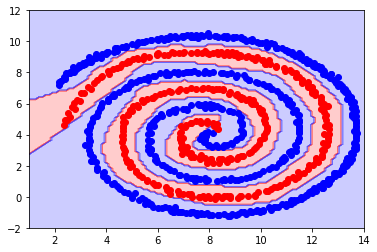## Week 5: Introduction to neural Networks¶

### Perceptron learning rule¶

This week, we will start working with neural networks. For each of the exercises below you can use the method of your choice but you should display the final boundary of your classifier.

#### Exercise 1.¶

As a first exercise, load the binary dataset below and code a few steps of the perceptron learning rule.

In :
#

import scipy.io as sio
data1 = sio.loadmat('perceptron_data_class1.mat')
data2 = sio.loadmat('perceptron_data_class2.mat')

from numpy import linalg as LA

data1 = data1['perceptron_data_class1']
data2 = data2['perceptron_data_class2']

# We first build the matrix of features (here [1, x, y])

sz1 = np.shape(data1)
sz2 = np.shape(data2)

targetsClass1 = np.ones((sz1,))
targetsClass2 = -1 * np.ones((sz2,))

total_targets = np.hstack((targetsClass1, targetsClass2))
total_data = np.vstack((data1, data2))

# precomputing the product y(x_i)t_i
product_yiti = np.multiply(np.vstack((data1, data2)),(np.ones((2,1))* total_targets).T)

total_Xtilde = np.hstack((np.ones((np.shape(total_data),1)), total_data))

# Then we initialize the beta_tilde (here I chose to take beta_tilde random Gaussian but any other choice is also possible)

sigma = 1
beta = np.random.normal(0, 1, (2,))
beta0 = np.random.normal(0, 1, 1)

beta_tilde_int = np.hstack((beta0, beta))
betaTotal = beta_tilde_int

# Initialization of the max number of iter and learning rate
eta = .01
iter_num = 1
max_iter = 100

while iter_num < 200:

# We start by looking for the misclassified points (in the case of the perceptron,
# the misclassified points are the points for which the sign of the product y(x_i)t_i is negative)

sign = np.sign(np.multiply(np.matmul(betaTotal,total_Xtilde.T),total_targets))
ind_misclassified = np.where(sign < 0)
misclassified_targets = total_targets[sign < 0]
# we then extract the misclassified products $x_it_i$
misclassified_yiti = product_yiti[sign < 0,:]
# now summing each of the misclassified vectors to get the gradient, we get
gradient_beta0 = np.sum(misclassified_targets, axis=0)
gradient_beta = np.sum(misclassified_yiti, axis=0)
gradient_betaTotal = np.hstack((gradient_beta0, gradient_beta))
print('gradient')
print(gradient_betaTotal)
print('norm of gradient')
print(LA.norm(gradient_betaTotal))
if LA.norm(gradient_betaTotal)!=0:
gradient_betaTotal = np.true_divide(gradient_betaTotal,LA.norm(gradient_betaTotal))

print(gradient_beta)

# updating Beta with learning rate eta
betaTotal += gradient_betaTotal * eta

iter_num +=1
print(iter_num)

gradient
[ 22.         372.75345622 793.64963504]
norm of gradient
877.1025494880358
[372.75345622 793.64963504]
2
gradient
[ 22.         372.75345622 793.64963504]
norm of gradient
877.1025494880358
[372.75345622 793.64963504]
3
gradient
[ 22.         372.75345622 793.64963504]
norm of gradient
877.1025494880358
[372.75345622 793.64963504]
4
gradient
[ 22.         372.75345622 793.64963504]
norm of gradient
877.1025494880358
[372.75345622 793.64963504]
5
gradient
[ 22.         372.75345622 793.64963504]
norm of gradient
877.1025494880358
[372.75345622 793.64963504]
6
gradient
[ 22.         372.75345622 793.64963504]
norm of gradient
877.1025494880358
[372.75345622 793.64963504]
7
gradient
[ 22.         372.75345622 793.64963504]
norm of gradient
877.1025494880358
[372.75345622 793.64963504]
8
gradient
[ 22.         372.75345622 793.64963504]
norm of gradient
877.1025494880358
[372.75345622 793.64963504]
9
gradient
[ 22.         372.75345622 793.64963504]
norm of gradient
877.1025494880358
[372.75345622 793.64963504]
10
gradient
[ 22.         372.75345622 793.64963504]
norm of gradient
877.1025494880358
[372.75345622 793.64963504]
11
gradient
[ 22.         372.75345622 793.64963504]
norm of gradient
877.1025494880358
[372.75345622 793.64963504]
12
gradient
[ 22.         372.75345622 793.64963504]
norm of gradient
877.1025494880358
[372.75345622 793.64963504]
13
gradient
[ 22.         372.75345622 793.64963504]
norm of gradient
877.1025494880358
[372.75345622 793.64963504]
14
gradient
[ 22.         372.75345622 793.64963504]
norm of gradient
877.1025494880358
[372.75345622 793.64963504]
15
gradient
[ 22.         372.75345622 793.64963504]
norm of gradient
877.1025494880358
[372.75345622 793.64963504]
16
gradient
[ 22.         372.75345622 793.64963504]
norm of gradient
877.1025494880358
[372.75345622 793.64963504]
17
gradient
[ 22.         372.75345622 793.64963504]
norm of gradient
877.1025494880358
[372.75345622 793.64963504]
18
gradient
[ 22.         372.75345622 793.64963504]
norm of gradient
877.1025494880358
[372.75345622 793.64963504]
19
gradient
[ 22.         372.75345622 793.64963504]
norm of gradient
877.1025494880358
[372.75345622 793.64963504]
20
gradient
[ 22.         372.75345622 793.64963504]
norm of gradient
877.1025494880358
[372.75345622 793.64963504]
21
gradient
[ 22.         372.75345622 793.64963504]
norm of gradient
877.1025494880358
[372.75345622 793.64963504]
22
gradient
[ 22.         372.75345622 793.64963504]
norm of gradient
877.1025494880358
[372.75345622 793.64963504]
23
gradient
[ 22.         372.75345622 793.64963504]
norm of gradient
877.1025494880358
[372.75345622 793.64963504]
24
gradient
[ 22.         372.75345622 793.64963504]
norm of gradient
877.1025494880358
[372.75345622 793.64963504]
25
gradient
[ 22.         372.75345622 793.64963504]
norm of gradient
877.1025494880358
[372.75345622 793.64963504]
26
gradient
[ 22.         372.75345622 793.64963504]
norm of gradient
877.1025494880358
[372.75345622 793.64963504]
27
gradient
[ 22.         372.75345622 793.64963504]
norm of gradient
877.1025494880358
[372.75345622 793.64963504]
28
gradient
[ 22.         372.75345622 793.64963504]
norm of gradient
877.1025494880358
[372.75345622 793.64963504]
29
gradient
[ 22.         372.75345622 793.64963504]
norm of gradient
877.1025494880358
[372.75345622 793.64963504]
30
gradient
[ 22.         372.75345622 793.64963504]
norm of gradient
877.1025494880358
[372.75345622 793.64963504]
31
gradient
[ 22.         372.75345622 793.64963504]
norm of gradient
877.1025494880358
[372.75345622 793.64963504]
32
gradient
[ 22.         372.75345622 793.64963504]
norm of gradient
877.1025494880358
[372.75345622 793.64963504]
33
gradient
[ 22.         372.75345622 793.64963504]
norm of gradient
877.1025494880358
[372.75345622 793.64963504]
34
gradient
[ 22.         372.75345622 793.64963504]
norm of gradient
877.1025494880358
[372.75345622 793.64963504]
35
gradient
[ 22.         372.75345622 793.64963504]
norm of gradient
877.1025494880358
[372.75345622 793.64963504]
36
gradient
[ 22.         372.75345622 793.64963504]
norm of gradient
877.1025494880358
[372.75345622 793.64963504]
37
gradient
[ 22.         372.75345622 793.64963504]
norm of gradient
877.1025494880358
[372.75345622 793.64963504]
38
gradient
[ 22.         372.75345622 793.64963504]
norm of gradient
877.1025494880358
[372.75345622 793.64963504]
39
gradient
[ 22.         372.75345622 793.64963504]
norm of gradient
877.1025494880358
[372.75345622 793.64963504]
40
gradient
[ 22.         372.75345622 793.64963504]
norm of gradient
877.1025494880358
[372.75345622 793.64963504]
41
gradient
[ 22.         372.75345622 793.64963504]
norm of gradient
877.1025494880358
[372.75345622 793.64963504]
42
gradient
[ 22.         372.75345622 793.64963504]
norm of gradient
877.1025494880358
[372.75345622 793.64963504]
43
gradient
[ 22.         372.75345622 793.64963504]
norm of gradient
877.1025494880358
[372.75345622 793.64963504]
44
gradient
[ 22.         372.75345622 793.64963504]
norm of gradient
877.1025494880358
[372.75345622 793.64963504]
45
gradient
[ 22.         372.75345622 793.64963504]
norm of gradient
877.1025494880358
[372.75345622 793.64963504]
46
gradient
[ 22.         372.75345622 793.64963504]
norm of gradient
877.1025494880358
[372.75345622 793.64963504]
47
gradient
[ 22.         372.75345622 793.64963504]
norm of gradient
877.1025494880358
[372.75345622 793.64963504]
48
gradient
[ 22.         372.75345622 793.64963504]
norm of gradient
877.1025494880358
[372.75345622 793.64963504]
49
gradient
[ 22.         372.75345622 793.64963504]
norm of gradient
877.1025494880358
[372.75345622 793.64963504]
50
gradient
[ 22.         372.75345622 793.64963504]
norm of gradient
877.1025494880358
[372.75345622 793.64963504]
51
gradient
[ 21.         345.01728111 790.69343066]
norm of gradient
862.9449724900206
[345.01728111 790.69343066]
52
gradient
[ 20.         327.07373272 787.88321168]
norm of gradient
853.3095463429563
[327.07373272 787.88321168]
53
gradient
[ 20.         327.07373272 787.88321168]
norm of gradient
853.3095463429563
[327.07373272 787.88321168]
54
gradient
[ 19.         293.23156682 783.46715328]
norm of gradient
836.7595425544075
[293.23156682 783.46715328]
55
gradient
[ 19.         293.23156682 783.46715328]
norm of gradient
836.7595425544075
[293.23156682 783.46715328]
56
gradient
[ 19.         293.23156682 783.46715328]
norm of gradient
836.7595425544075
[293.23156682 783.46715328]
57
gradient
[ 18.         253.28341014 776.86131387]
norm of gradient
817.3065439826896
[253.28341014 776.86131387]
58
gradient
[ 18.         253.28341014 776.86131387]
norm of gradient
817.3065439826896
[253.28341014 776.86131387]
59
gradient
[ 18.         253.28341014 776.86131387]
norm of gradient
817.3065439826896
[253.28341014 776.86131387]
60
gradient
[ 17.         214.25691244 768.50364964]
norm of gradient
797.9930350771989
[214.25691244 768.50364964]
61
gradient
[ 16.         192.62672811 761.89781022]
norm of gradient
786.0339239492583
[192.62672811 761.89781022]
62
gradient
[ 16.         192.62672811 761.89781022]
norm of gradient
786.0339239492583
[192.62672811 761.89781022]
63
gradient
[ 14.         110.65668203 739.34306569]
norm of gradient
747.7092149133439
[110.65668203 739.34306569]
64
gradient
[ 12.          41.359447   717.08029197]
norm of gradient
718.372291356967
[ 41.359447   717.08029197]
65
gradient
[ 10.         -15.72580645 696.71532847]
norm of gradient
696.9645255747577
[-15.72580645 696.71532847]
66
gradient
[ 10.         -15.72580645 696.71532847]
norm of gradient
696.9645255747577
[-15.72580645 696.71532847]
67
gradient
[ 10.         -15.72580645 696.71532847]
norm of gradient
696.9645255747577
[-15.72580645 696.71532847]
68
gradient
[ 10.         -15.72580645 696.71532847]
norm of gradient
696.9645255747577
[-15.72580645 696.71532847]
69
gradient
[  9.         -28.25460829 688.94160584]
norm of gradient
689.5794799343245
[-28.25460829 688.94160584]
70
gradient
[  9.         -28.25460829 688.94160584]
norm of gradient
689.5794799343245
[-28.25460829 688.94160584]
71
gradient
[  8.         -71.42857143 671.09489051]
norm of gradient
674.9328802823502
[-71.42857143 671.09489051]
72
gradient
[   6.         -130.5875576   641.38686131]
norm of gradient
654.5733083978846
[-130.5875576   641.38686131]
73
gradient
[   3.         -228.54262673  592.66423358]
norm of gradient
635.209907032922
[-228.54262673  592.66423358]
74
gradient
[   3.         -228.54262673  592.66423358]
norm of gradient
635.209907032922
[-228.54262673  592.66423358]
75
gradient
[   3.         -228.54262673  592.66423358]
norm of gradient
635.209907032922
[-228.54262673  592.66423358]
76
gradient
[   3.         -228.54262673  592.66423358]
norm of gradient
635.209907032922
[-228.54262673  592.66423358]
77
gradient
[   3.         -228.54262673  592.66423358]
norm of gradient
635.209907032922
[-228.54262673  592.66423358]
78
gradient
[   2.         -257.89170507  574.81751825]
norm of gradient
630.0216748878181
[-257.89170507  574.81751825]
79
gradient
[   2.         -257.89170507  574.81751825]
norm of gradient
630.0216748878181
[-257.89170507  574.81751825]
80
gradient
[   2.         -257.89170507  574.81751825]
norm of gradient
630.0216748878181
[-257.89170507  574.81751825]
81
gradient
[   1.         -263.39285714  560.91240876]
norm of gradient
619.6769541413013
[-263.39285714  560.91240876]
82
gradient
[   0.         -287.32718894  541.60583942]
norm of gradient
613.1017850192484
[-287.32718894  541.60583942]
83
gradient
[  -2.         -300.63364055  505.62043796]
norm of gradient
588.2487680490048
[-300.63364055  505.62043796]
84
gradient
[  -4.         -359.10138249  459.41605839]
norm of gradient
583.1234154238397
[-359.10138249  459.41605839]
85
gradient
[  -6.         -390.61059908  410.87591241]
norm of gradient
566.94942941154
[-390.61059908  410.87591241]
86
gradient
[  -4.         -250.69124424  425.10948905]
norm of gradient
493.5384256769653
[-250.69124424  425.10948905]
87
gradient
[  -8.         -215.43778802  191.97080292]
norm of gradient
288.6697588593456
[-215.43778802  191.97080292]
88
gradient
[ -4.         -34.33179724 150.3649635 ]
norm of gradient
154.28640429681616
[-34.33179724 150.3649635 ]
89
gradient
[ -13.         -316.33064516 -163.39416058]
norm of gradient
356.2747939176852
[-316.33064516 -163.39416058]
90
gradient
[  1.          98.58870968 219.30656934]
norm of gradient
240.44979732343998
[ 98.58870968 219.30656934]
91
gradient
[ -11.         -255.78917051 -144.19708029]
norm of gradient
293.8399185157514
[-255.78917051 -144.19708029]
92
gradient
[  3.         141.96428571 238.21167883]
norm of gradient
277.32230770419983
[141.96428571 238.21167883]
93
gradient
[ -10.         -228.05299539 -134.52554745]
norm of gradient
264.96281177285
[-228.05299539 -134.52554745]
94
gradient
[  3.         141.96428571 238.21167883]
norm of gradient
277.32230770419983
[141.96428571 238.21167883]
95
gradient
[  -9.         -198.70391705 -116.67883212]
norm of gradient
230.60398200253536
[-198.70391705 -116.67883212]
96
gradient
[  4.         159.9078341  241.02189781]
norm of gradient
289.27162085319554
[159.9078341  241.02189781]
97
gradient
[  -8.         -168.20276498  -93.43065693]
norm of gradient
192.57584947513544
[-168.20276498  -93.43065693]
98
gradient
[  3.         132.40207373 197.48175182]
norm of gradient
237.77794563946242
[132.40207373 197.48175182]
99
gradient
[  -6.         -121.02534562  -72.77372263]
norm of gradient
141.34761755350667
[-121.02534562  -72.77372263]
100
gradient
[  2.         102.24654378 151.16788321]
norm of gradient
182.51050553162744
[102.24654378 151.16788321]
101
gradient
[  -6.         -121.02534562  -72.77372263]
norm of gradient
141.34761755350667
[-121.02534562  -72.77372263]
102
gradient
[  1.          74.04953917 112.15328467]
norm of gradient
134.3975204904403
[ 74.04953917 112.15328467]
103
gradient
[  -5.         -103.08179724  -69.96350365]
norm of gradient
124.68259206535166
[-103.08179724  -69.96350365]
104
gradient
[  2.         90.9562212 119.7810219]
norm of gradient
150.41451851976154
[ 90.9562212 119.7810219]
105
gradient
[  -5.         -103.08179724  -69.96350365]
norm of gradient
124.68259206535166
[-103.08179724  -69.96350365]
106
gradient
[  2.         90.9562212 119.7810219]
norm of gradient
150.41451851976154
[ 90.9562212 119.7810219]
107
gradient
[  -5.         -103.08179724  -69.96350365]
norm of gradient
124.68259206535166
[-103.08179724  -69.96350365]
108
gradient
[  2.         90.9562212 119.7810219]
norm of gradient
150.41451851976154
[ 90.9562212 119.7810219]
109
gradient
[  -5.         -103.08179724  -69.96350365]
norm of gradient
124.68259206535166
[-103.08179724  -69.96350365]
110
gradient
[  2.         90.9562212 119.7810219]
norm of gradient
150.41451851976154
[ 90.9562212 119.7810219]
111
gradient
[ -4.         -81.33640553 -57.66423358]
norm of gradient
99.78363943309886
[-81.33640553 -57.66423358]
112
gradient
[ 1.         58.03571429 75.51094891]
norm of gradient
95.2420471073733
[58.03571429 75.51094891]
113
gradient
[ -4.         -81.33640553 -57.66423358]
norm of gradient
99.78363943309886
[-81.33640553 -57.66423358]
114
gradient
[ 1.         58.03571429 75.51094891]
norm of gradient
95.2420471073733
[58.03571429 75.51094891]
115
gradient
[ -4.         -81.33640553 -57.66423358]
norm of gradient
99.78363943309886
[-81.33640553 -57.66423358]
116
gradient
[ 1.         58.03571429 75.51094891]
norm of gradient
95.2420471073733
[58.03571429 75.51094891]
117
gradient
[ -3.         -53.36981567 -34.7080292 ]
norm of gradient
63.73369999618191
[-53.36981567 -34.7080292 ]
118
gradient
[ 1.         58.03571429 75.51094891]
norm of gradient
95.2420471073733
[58.03571429 75.51094891]
119
gradient
[ -3.         -53.36981567 -34.7080292 ]
norm of gradient
63.73369999618191
[-53.36981567 -34.7080292 ]
120
gradient
[ 1.         58.03571429 75.51094891]
norm of gradient
95.2420471073733
[58.03571429 75.51094891]
121
gradient
[ -2.         -36.46313364 -27.08029197]
norm of gradient
45.4631975130738
[-36.46313364 -27.08029197]
122
gradient
[ 1.         58.03571429 75.51094891]
norm of gradient
95.2420471073733
[58.03571429 75.51094891]
123
gradient
[ -2.         -36.46313364 -27.08029197]
norm of gradient
45.4631975130738
[-36.46313364 -27.08029197]
124
gradient
[ 1.         58.03571429 75.51094891]
norm of gradient
95.2420471073733
[58.03571429 75.51094891]
125
gradient
[ -2.         -36.46313364 -27.08029197]
norm of gradient
45.4631975130738
[-36.46313364 -27.08029197]
126
gradient
[ 1.         58.03571429 75.51094891]
norm of gradient
95.2420471073733
[58.03571429 75.51094891]
127
gradient
[ -2.         -36.46313364 -27.08029197]
norm of gradient
45.4631975130738
[-36.46313364 -27.08029197]
128
gradient
[ 1.         58.03571429 75.51094891]
norm of gradient
95.2420471073733
[58.03571429 75.51094891]
129
gradient
[ -2.         -36.46313364 -27.08029197]
norm of gradient
45.4631975130738
[-36.46313364 -27.08029197]
130
gradient
[ 1.         58.03571429 75.51094891]
norm of gradient
95.2420471073733
[58.03571429 75.51094891]
131
gradient
[ -2.         -36.46313364 -27.08029197]
norm of gradient
45.4631975130738
[-36.46313364 -27.08029197]
132
gradient
[ 1.         58.03571429 75.51094891]
norm of gradient
95.2420471073733
[58.03571429 75.51094891]
133
gradient
[ -2.         -36.46313364 -27.08029197]
norm of gradient
45.4631975130738
[-36.46313364 -27.08029197]
134
gradient
[ 1.         58.03571429 75.51094891]
norm of gradient
95.2420471073733
[58.03571429 75.51094891]
135
gradient
[ -2.         -36.46313364 -27.08029197]
norm of gradient
45.4631975130738
[-36.46313364 -27.08029197]
136
gradient
[ 0.         24.07834101 35.18248175]
norm of gradient
42.633009842087475
[24.07834101 35.18248175]
137
gradient
[ -2.         -36.46313364 -27.08029197]
norm of gradient
45.4631975130738
[-36.46313364 -27.08029197]
138
gradient
[ 0.         24.07834101 35.18248175]
norm of gradient
42.633009842087475
[24.07834101 35.18248175]
139
gradient
[ -2.         -36.46313364 -27.08029197]
norm of gradient
45.4631975130738
[-36.46313364 -27.08029197]
140
gradient
[ 0.         24.07834101 35.18248175]
norm of gradient
42.633009842087475
[24.07834101 35.18248175]
141
gradient
[ -1.         -12.52880184  -7.77372263]
norm of gradient
14.778418018249504
[-12.52880184  -7.77372263]
142
gradient
[ 0.         24.07834101 35.18248175]
norm of gradient
42.633009842087475
[24.07834101 35.18248175]
143
gradient
[ -1.         -12.52880184  -7.77372263]
norm of gradient
14.778418018249504
[-12.52880184  -7.77372263]
144
gradient
[ 0.         24.07834101 35.18248175]
norm of gradient
42.633009842087475
[24.07834101 35.18248175]
145
gradient
[ -1.         -12.52880184  -7.77372263]
norm of gradient
14.778418018249504
[-12.52880184  -7.77372263]
146
gradient
[ -1.         -12.52880184  -7.77372263]
norm of gradient
14.778418018249504
[-12.52880184  -7.77372263]
147
gradient
[  3.         103.48502304 127.55474453]
norm of gradient
164.28135269978821
[103.48502304 127.55474453]
148
gradient
[ -1.         -12.52880184  -7.77372263]
norm of gradient
14.778418018249504
[-12.52880184  -7.77372263]
149
gradient
[ 2.         70.56451613 83.28467153]
norm of gradient
109.17732112878855
[70.56451613 83.28467153]
150
gradient
[ -1.         -12.52880184  -7.77372263]
norm of gradient
14.778418018249504
[-12.52880184  -7.77372263]
151
gradient
[ 2.         70.56451613 83.28467153]
norm of gradient
109.17732112878855
[70.56451613 83.28467153]
152
gradient
[ -1.         -12.52880184  -7.77372263]
norm of gradient
14.778418018249504
[-12.52880184  -7.77372263]
153
gradient
[ 2.         70.56451613 83.28467153]
norm of gradient
109.17732112878855
[70.56451613 83.28467153]
154
gradient
[ -1.         -12.52880184  -7.77372263]
norm of gradient
14.778418018249504
[-12.52880184  -7.77372263]
155
gradient
[ 2.         70.56451613 83.28467153]
norm of gradient
109.17732112878855
[70.56451613 83.28467153]
156
gradient
[ -1.         -12.52880184  -7.77372263]
norm of gradient
14.778418018249504
[-12.52880184  -7.77372263]
157
gradient
[ 2.         70.56451613 83.28467153]
norm of gradient
109.17732112878855
[70.56451613 83.28467153]
158
gradient
[0. 0. 0.]
norm of gradient
0.0
[0. 0.]
159
gradient
[0. 0. 0.]
norm of gradient
0.0
[0. 0.]
160
gradient
[0. 0. 0.]
norm of gradient
0.0
[0. 0.]
161
gradient
[0. 0. 0.]
norm of gradient
0.0
[0. 0.]
162
gradient
[0. 0. 0.]
norm of gradient
0.0
[0. 0.]
163
gradient
[0. 0. 0.]
norm of gradient
0.0
[0. 0.]
164
gradient
[0. 0. 0.]
norm of gradient
0.0
[0. 0.]
165
gradient
[0. 0. 0.]
norm of gradient
0.0
[0. 0.]
166
gradient
[0. 0. 0.]
norm of gradient
0.0
[0. 0.]
167
gradient
[0. 0. 0.]
norm of gradient
0.0
[0. 0.]
168
gradient
[0. 0. 0.]
norm of gradient
0.0
[0. 0.]
169
gradient
[0. 0. 0.]
norm of gradient
0.0
[0. 0.]
170
gradient
[0. 0. 0.]
norm of gradient
0.0
[0. 0.]
171
gradient
[0. 0. 0.]
norm of gradient
0.0
[0. 0.]
172
gradient
[0. 0. 0.]
norm of gradient
0.0
[0. 0.]
173
gradient
[0. 0. 0.]
norm of gradient
0.0
[0. 0.]
174
gradient
[0. 0. 0.]
norm of gradient
0.0
[0. 0.]
175
gradient
[0. 0. 0.]
norm of gradient
0.0
[0. 0.]
176
gradient
[0. 0. 0.]
norm of gradient
0.0
[0. 0.]
177
gradient
[0. 0. 0.]
norm of gradient
0.0
[0. 0.]
178
gradient
[0. 0. 0.]
norm of gradient
0.0
[0. 0.]
179
gradient
[0. 0. 0.]
norm of gradient
0.0
[0. 0.]
180
gradient
[0. 0. 0.]
norm of gradient
0.0
[0. 0.]
181
gradient
[0. 0. 0.]
norm of gradient
0.0
[0. 0.]
182
gradient
[0. 0. 0.]
norm of gradient
0.0
[0. 0.]
183
gradient
[0. 0. 0.]
norm of gradient
0.0
[0. 0.]
184
gradient
[0. 0. 0.]
norm of gradient
0.0
[0. 0.]
185
gradient
[0. 0. 0.]
norm of gradient
0.0
[0. 0.]
186
gradient
[0. 0. 0.]
norm of gradient
0.0
[0. 0.]
187
gradient
[0. 0. 0.]
norm of gradient
0.0
[0. 0.]
188
gradient
[0. 0. 0.]
norm of gradient
0.0
[0. 0.]
189
gradient
[0. 0. 0.]
norm of gradient
0.0
[0. 0.]
190
gradient
[0. 0. 0.]
norm of gradient
0.0
[0. 0.]
191
gradient
[0. 0. 0.]
norm of gradient
0.0
[0. 0.]
192
gradient
[0. 0. 0.]
norm of gradient
0.0
[0. 0.]
193
gradient
[0. 0. 0.]
norm of gradient
0.0
[0. 0.]
194
gradient
[0. 0. 0.]
norm of gradient
0.0
[0. 0.]
195
gradient
[0. 0. 0.]
norm of gradient
0.0
[0. 0.]
196
gradient
[0. 0. 0.]
norm of gradient
0.0
[0. 0.]
197
gradient
[0. 0. 0.]
norm of gradient
0.0
[0. 0.]
198
gradient
[0. 0. 0.]
norm of gradient
0.0
[0. 0.]
199
gradient
[0. 0. 0.]
norm of gradient
0.0
[0. 0.]
200

In :
import numpy as np

# we now plot the classification

xx, yy = np.meshgrid(np.linspace(0,50,100),
np.linspace(0,50,100))

tmp = np.array([xx.ravel(), yy.ravel()]).T
tmp1 = np.ones((np.shape(tmp),1))

phi_tilde = np.hstack((tmp1, tmp))

import matplotlib.pyplot as plt

C = np.array([ 'Red','Blue'])

Z = np.matmul(betaTotal, phi_tilde.T)
Z = Z.reshape(xx.shape)
plt.contourf(xx, yy, np.sign(Z), alpha=0.3, colors=C)

plt.scatter(data1[:,0], data1[:,1], facecolor='blue')
plt.scatter(data2[:,0], data2[:,1], facecolor='red')
plt.show()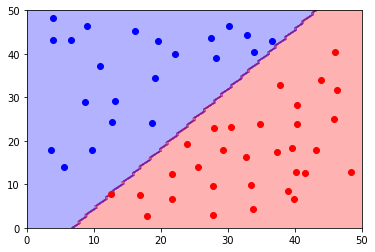#### Exercise 2.¶

2a. Load the data below. Using the neural_network module from scikit-learn and its MLPClassifier model, learn a classifier, for the dataset below using

• One hidden layer with a linear activation function and
• One neuron
• Two neurons
• One hidden layer with a non linear activation function (take Relu for example or a binary step)
• One neuron
• Two neurons

How many neurons, hidden layers do you need to learn the distribution of the data? Do you have an idea why?

Try increasing the number of neurons and hidden layers. Then try different values of the learning rate.

In :
## 1) This is the solution for the one neuron exercise
import scipy.io as sio
data1 = sio.loadmat('neural_net_class1.mat')
data2 = sio.loadmat('neural_net_class2.mat')

data1 = data1['neural_net_class1']
data2 = data2['neural_net_class2']

from sklearn.neural_network import MLPClassifier

# put your code here

import matplotlib.pyplot as plt
import numpy as np

sz1 = np.shape(data1)
sz2 = np.shape(data2)
targetsClass1 = np.ones((sz1,))
targetsClass2 = -1 * np.ones((sz2,))

total_targets = np.hstack((targetsClass1, targetsClass2))

total_data = np.vstack((data1, data2))

from sklearn.neural_network import MLPClassifier

my_classifier = MLPClassifier(hidden_layer_sizes = 1, activation = 'identity')

my_classifier.fit(total_data, total_targets)

from matplotlib.colors import ListedColormap
# plot the decision surface

colors = ('red', 'blue', 'lightgreen', 'gray', 'cyan')
cmap = ListedColormap(colors[:2])

xx, yy = np.meshgrid(np.linspace(0,50,100),
np.linspace(0,50,100))
Z = my_classifier.predict(np.array([xx.ravel(), yy.ravel()]).T)
Z = Z.reshape(xx.shape)
plt.contourf(xx, yy, Z, alpha=0.2, cmap=cmap)
plt.xlim(xx.min(), xx.max())
plt.ylim(yy.min(), yy.max())

plt.scatter(data1[:,0], data1[:,1], facecolor='blue')
plt.scatter(data2[:,0], data2[:,1], facecolor='red')
plt.show()

## 2) Adding a couple more neurons won't change the ouptut a lot.

172
195
(172,)
(195,)
(367,)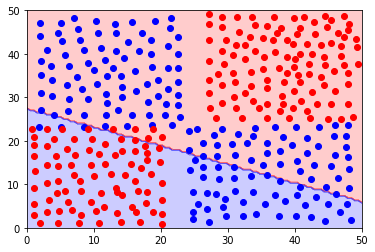In :
## 2) Using a non linear activation function

my_classifier = MLPClassifier(hidden_layer_sizes = (100,), activation = 'relu')

my_classifier.fit(total_data, total_targets)

from matplotlib.colors import ListedColormap
# plot the decision surface

colors = ('red', 'blue', 'lightgreen', 'gray', 'cyan')
cmap = ListedColormap(colors[:2])

xx, yy = np.meshgrid(np.linspace(0,50,100),
np.linspace(0,50,100))
Z = my_classifier.predict(np.array([xx.ravel(), yy.ravel()]).T)
Z = Z.reshape(xx.shape)
plt.contourf(xx, yy, Z, alpha=0.2, cmap=cmap)
plt.xlim(xx.min(), xx.max())
plt.ylim(yy.min(), yy.max())

plt.scatter(data1[:,0], data1[:,1], facecolor='blue')
plt.scatter(data2[:,0], data2[:,1], facecolor='red')
plt.show()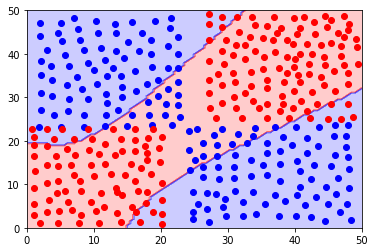In :
## 3) changing the learning rate
## Try various values for the learning rate between .0001 and 1. What do you observe ?

my_classifier = MLPClassifier(hidden_layer_sizes = (20,20,20), activation = 'relu', learning_rate  = 'constant', learning_rate_init =.001, max_iter=20000)

my_classifier.fit(total_data, total_targets)

from matplotlib.colors import ListedColormap
# plot the decision surface

colors = ('red', 'blue', 'lightgreen', 'gray', 'cyan')
cmap = ListedColormap(colors[:2])

xx, yy = np.meshgrid(np.linspace(0,50,100),
np.linspace(0,50,100))
Z = my_classifier.predict(np.array([xx.ravel(), yy.ravel()]).T)
Z = Z.reshape(xx.shape)
plt.contourf(xx, yy, Z, alpha=0.2, cmap=cmap)
plt.xlim(xx.min(), xx.max())
plt.ylim(yy.min(), yy.max())

plt.scatter(data1[:,0], data1[:,1], facecolor='blue')
plt.scatter(data2[:,0], data2[:,1], facecolor='red')
plt.show()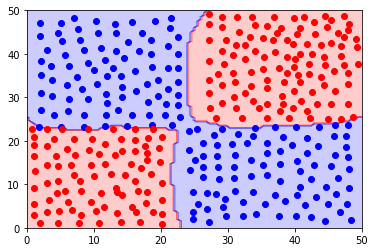2b. Keep the dataset from above. try to change the intialization of the training algorithm. Plot the resulting classifier for a couple of different initializations. What do you see?

Do it for a small network first. Then repeat those experiments for larger architectures. I.e. increase the number of neurons and the number of layers. What do you see when you change the initialization?

In :
# Although it is a little more tricky, it is possible to use object oriented programming and inheritance to modify
# the _init_coef method from the MLPClassifier class in scikit learn. As an illustration of this, in the example below,
# we replace the built-in initialization of the MLP class and replace the initial weights by zeros.

from sklearn.neural_network import MLPClassifier

class MLPClassifierOverride(MLPClassifier):

def _init_coef(self, fan_in, fan_out):

if self.activation == 'logistic':

init_bound = np.sqrt(2. / (fan_in + fan_out))

elif self.activation in ('identity', 'tanh', 'relu'):

init_bound = np.sqrt(6. / (fan_in + fan_out))

else:

raise ValueError("Unknown activation function %s" %
self.activation)
coef_init = np.zeros(np.shape(self._random_state.uniform(-init_bound, init_bound,
(fan_in, fan_out))))

intercept_init = np.zeros(np.shape(self._random_state.uniform(-init_bound, init_bound,
fan_out)))
return coef_init, intercept_init

## Try various values for the learning rate between .0001 and 1. What do you observe ?

import scipy.io as sio
data1 = sio.loadmat('neural_net_class1.mat')
data2 = sio.loadmat('neural_net_class2.mat')

data1 = data1['neural_net_class1']
data2 = data2['neural_net_class2']

from sklearn.neural_network import MLPClassifier

# put your code here

import matplotlib.pyplot as plt
import numpy as np

sz1 = np.shape(data1)
sz2 = np.shape(data2)
targetsClass1 = np.ones((sz1,))
targetsClass2 = -1 * np.ones((sz2,))

total_targets = np.hstack((targetsClass1, targetsClass2))

total_data = np.vstack((data1, data2))

my_classifier = MLPClassifier(hidden_layer_sizes = (100,), activation = 'relu', learning_rate  = 'constant', learning_rate_init =.001)

my_classifier.fit(total_data, total_targets)

from matplotlib.colors import ListedColormap
## plot the decision surface

colors = ('red', 'blue', 'lightgreen', 'gray', 'cyan')
cmap = ListedColormap(colors[:2])

xx, yy = np.meshgrid(np.linspace(0,50,100),
np.linspace(0,50,100))
Z = my_classifier.predict(np.array([xx.ravel(), yy.ravel()]).T)
Z = Z.reshape(xx.shape)
plt.contourf(xx, yy, Z, alpha=0.2, cmap=cmap)
plt.xlim(xx.min(), xx.max())
plt.ylim(yy.min(), yy.max())

plt.scatter(data1[:,0], data1[:,1], facecolor='blue')
plt.scatter(data2[:,0], data2[:,1], facecolor='red')
plt.show()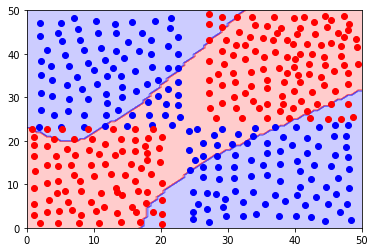#### Exercise 3.¶

3a.Load the data below. Try to build the best neural network you can for this dataset. Split the data between a training and a test set and evaluate the models you built. What is the best validation error you can get?

In :
# The spiral is relatively hard because of its strong non-linearity. However, as shown below,
# it remains of course possible to fit it with a neural network provided that (1) the network is sufficiently over-parametrized
# (i.e. taking a sufficiently large number of layers and neurons in each layer) and (2) that one takes a sufficiently
# small learning with a (batch) gradient descent algorithm (taking all the samples into account, thus avoiding any randomness in the iterations).
# To avoid disproportionate complexity of the classifier, it is also good to set the regularization parameter to some relatively
# large constant, here I choose alpha = .1

import scipy.io as sio
data1 = sio.loadmat('neural_net_ex2_class1.mat')
data2 = sio.loadmat('neural_net_ex2_class2.mat')

data1 = data1['neural_net_ex2_class1']
data2 = data2['neural_net_ex2_class2']

from sklearn.neural_network import MLPClassifier

# put your code here

import matplotlib.pyplot as plt
import numpy as np

sz1 = np.shape(data1)
sz2 = np.shape(data2)
targetsClass1 = np.ones((sz1,))
targetsClass2 = -1 * np.ones((sz2,))

total_targets = np.hstack((targetsClass1, targetsClass2))
total_data = np.vstack((data1, data2))

from sklearn.neural_network import MLPClassifier

## We first try a simple MLP with Relu activation and without any additional features

my_classifier = MLPClassifier(hidden_layer_sizes = (100,100), activation = 'tanh', max_iter=40000, solver = 'lbfgs', alpha = .1)

my_classifier.fit(total_data, total_targets)

from matplotlib.colors import ListedColormap
# plot the decision surface

colors = ('red', 'blue', 'lightgreen', 'gray', 'cyan')
cmap = ListedColormap(colors[:2])

xx, yy = np.meshgrid(np.linspace(0,50,100),
np.linspace(0,50,100))
Z = my_classifier.predict(np.array([xx.ravel(), yy.ravel()]).T)
Z = Z.reshape(xx.shape)
plt.contourf(xx, yy, Z, alpha=0.2, cmap=cmap)

plt.scatter(data1[:,0], data1[:,1], facecolor='blue')
plt.scatter(data2[:,0], data2[:,1], facecolor='red')
plt.show()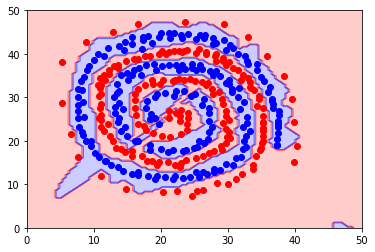3b. With the same dataset, add additional features to your model, e.g. $\sin(x), \sin(y)$ or other monomials. Can you improve your classifier ?

In [ ]:
# Even when using Neural networks, it might be interesting to add additional features. Not because the network won't
# be able to learn the classifier, in fact we know from the Universal approximation Theorem that a sufficiently large
# Perceptron can learn any distribution, but because adding a couple of features such as sin(x), cos(x) and x^2, y^2
# might lead to a simpler architecture. Random initialization however makes the learning tricky.


Why is the spiral example so difficult to learn?

In :
## 1) Once again, the difficulty for the spiral comes from the strong non linearity of the data. Such a non linearity
# requires a sufficiently large architecture and some relatively good intuition on the way neural networks can capture a
# distribution. However, as shown below on yet another example, neural networks are perfectly able to recover a
# relatively regular boundary even on such strongly non linear dataset.

import numpy as np
import scipy.io as sio
data1 = sio.loadmat('pointsSpiralClass1_1.mat')
data2 = sio.loadmat('pointsSpiralClass1_2.mat')
data3 = sio.loadmat('pointsSpiralClass2_1.mat')

data1 = data1['pointsSpiralClass1_1']
data2 = data2['pointsSpiralClass1_2']
data3 = data3['pointsSpiralClass2_1']

data1 = np.vstack((data1,data2))
print(np.shape(data1))

sz1 = np.shape(data1)
sz2 = np.shape(data3)

targets_class1 = np.ones((sz1,))
targets_class2 = -1*np.ones((sz2,))

total_data = np.vstack((data1, data3))
total_targets = np.hstack((targets_class1, targets_class2))

from sklearn.neural_network import MLPClassifier

## We first try a simple MLP with Relu activation and without any additional features

my_classifier = MLPClassifier(hidden_layer_sizes = (100,100), activation = 'tanh', max_iter=10000, batch_size=1000,learning_rate_init=0.001)

my_classifier.fit(total_data, total_targets)

from matplotlib.colors import ListedColormap

colors = ('red', 'blue', 'lightgreen', 'gray', 'cyan')
cmap = ListedColormap(colors[:2])

xx, yy = np.meshgrid(np.linspace(1,14,100),
np.linspace(-2,12,100))

preprocessed = np.array([xx.ravel(), yy.ravel()]).T

# from sklearn.preprocessing import PolynomialFeatures
# poly = PolynomialFeatures(2)
# preprocessed = poly.fit_transform(preprocessed)
# preprocessed = preprocessed[:,1:]
# preprocessed = np.hstack((preprocessed,np.sin(preprocessed)) )

Z = my_classifier.predict(preprocessed)
Z = Z.reshape(xx.shape)

import matplotlib.pyplot as plt
plt.contourf(xx, yy, Z, alpha=0.2, cmap=cmap)
plt.scatter(data1[:,0], data1[:,1], facecolor='blue')
plt.scatter(data3[:,0], data3[:,1], facecolor='red')
plt.xlim(xx.min(), xx.max())
plt.ylim(yy.min(), yy.max())
plt.show()

(557, 2)